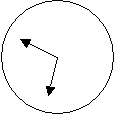#### You may also like### Diophantine N-tuples

Can you explain why a sequence of operations always gives you perfect squares?### DOTS Division

Take any pair of two digit numbers x=ab and y=cd where, without loss of generality, ab > cd . Form two 4 digit numbers r=abcd and s=cdab and calculate: {r^2 - s^2} /{x^2 - y^2}.### Sixational

The nth term of a sequence is given by the formula n^3 + 11n . Find the first four terms of the sequence given by this formula and the first term of the sequence which is bigger than one million. Prove that all terms of the sequence are divisible by 6.

# Hand Swap

##### Age 14 to 16Challenge Level

There were lots of approximate solutions here coming so close that the hands would appear to swop over. The closest were Selina Tang from The International School, Brunei who suggested that the train left at 6.47 and arrived at 9.34 taking 2 hours 47 minutes and Kang Hong Joo, The Chinese High School, Singapore, who suggested 2 hours 46 minutes but admitted to being unable to explain why. If you know that it leaves between 6.47 and 6.48 and arrives between 9.33 and 9.34 can you get closer to the exact times?As often seems to happen 3 solutions arrived almost simultaneously to this. Edwin Taylor , The Leventhorpe School, Sawbridgeworth, Herts, Ling Xiang Ning (who is doing great work in cracking many of these tough nuts) and Stephan Bach-Frommer and Steven Shaw from King George the Fifth School, Hong Kong are to be congratulated on their solutions.

What follows is Stephan's account of their work. The NRICH team was particularly pleased to receive this because it was not a method we had used for this problem. "This puzzle can be tackled in a number of different ways. At first I wrote the formula for the angular positions of both hands at a given time. However I soon realized that using an iterative approach would be somewhat faster. I solved the puzzle during break with my friend so I must mention him as well. I hope the solution is correct. As I said the question caught my interest because of the different number of approaches. I'm sure the one I chose was not the simplest but I was able to do the puzzle in about 15 minutes. Once again, great site and keep up the good work.

We formed the iterative formula by looking at the position of the hour and minute hands at a certain time. The position was defined as a fraction of the clock face so 45 minutes was equal to ${3\over 4}$.

By simply looking at a clock one can see that the time of embarkation must be after $6{\cdot}45$ but this time is taken as a first approximation to the starting time.

The hour hand moves $1/12$ of the fraction that the minute hand moves. The iteration depends on the fact that the position of the minute hand determines the position of the hour hand together with the fact that the two hands swop positions. Between six and seven o'clock the ${1\over 2}$ represents the initial six hours that the hour hand had moved previously.

When the minute hand is at $X$ (between 9 and 10 on the clock face) the hour hand is at $${X\over 12} + {1\over 2}$$ (between 6 and 7 on the clock face).

If, at the finish, the minute hand is at $${X\over 12} + {1\over2}$$ (between 6 and 7 on the clock face) the hour hand is at $${3\over4} + ({X\over 12} + {1\over 2}) \times {1\over 12}$$ (between 9 and 10 on the clock face). The iteration takes this as the new position of the minute hand at the start. \par To discover the iterative formula, we did the iteration `manually' for a few steps.

 Time Minute Hour $6{\cdot}45$ $\frac{3}{4}$ $\rightarrow$ $\frac{3}{4} \times \frac{1}{12}+\frac{1}{2}=\frac{9}{16}$ $\downarrow$ $9{\cdot}30$ $\frac{51}{64}\times\frac{1}{12}+\frac{1}{2}= \frac{145}{256}$ $\leftarrow$ $\frac{3}{4}+\frac{9}{16}\times\frac{1}{12}=\frac{51}{64}$

From the working above the formula can be seen to be: $$X_{n+1}=\left(\left({X_n\over 12}+{1\over 2}\right)\times{1\over 2} \right)+{3\over 4}, \quad X_0 = {3\over 4}.$$ A quick consultation with our calculators gives the starting position of the minute hand to be $114/143$. This corresponds to $$60 \times{114\over 143} = 47\frac{119}{143} = 47{\cdot}832167\ \mathrm{recurring\ minutes.}$$

The ending position of the minute hand is therefore $$\frac{114}{143}\times \frac{1}{12} + \frac{1}{2} = \frac{81}{143}.$$ This corresponds to $$33\frac{141}{143} = 33{\cdot}986013\ \mathrm{recurring\ minutes.}$$

The starting time is $$6\ {\rm hours} + 47\frac{119}{143}\ {\rm minutes}$$ and the finishing time is $$9\ {\rm hours} + 33\frac{141}{143}\ {\rm minutes}$$

Therefore the total time taken for the journey is $$2\ {\rm hours}+ 46\frac{22}{143}\ {\rm minutes}$$ that is 2 hours 46 minutes and 120/13 seconds or 2 hours 46 minutes 9 seconds to the nearest second.''

Footnote : Assuming the iteration given by the formula $$X_{n+1}=\left(\left({X_n\over 12}+{1\over 2}\right)\times{1\over 2} \right)+{3\over 4}$$ tends to a limit, say $L$, this limit can be found by solving the equation $$L=\left(\frac{L}{12}+\frac{1} {2}\right)\times\frac{1}{2}$$